Atmos. Chem. Phys., 19, 13581–13589, 2019
https://doi.org/10.5194/acp-19-13581-2019
Atmos. Chem. Phys., 19, 13581–13589, 2019
https://doi.org/10.5194/acp-19-13581-2019

Technical note 08 Nov 2019

Technical note | 08 Nov 2019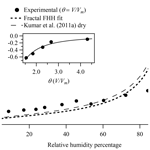# Technical note: Frenkel, Halsey and Hill analysis of water on clay minerals: toward closure between cloud condensation nuclei activity and water adsorption

Technical note: Frenkel, Halsey and Hill analysis of water on clay minerals: toward closure between cloud condensation nuclei activity and water adsorption
Courtney D. Hatch, Paul R. Tumminello, Megan A. Cassingham, Ann L. Greenaway, Rebecca Meredith, and Matthew J. Christie Courtney D. Hatch et al.
• Department of Chemistry, Hendrix College, 1600 Washington Ave., Conway, AR, 72032, USA

Correspondence: Courtney D. Hatch (hatch@hendrix.edu)

Abstract

Insoluble atmospheric aerosol, such as mineral dust, has been identified as an important contributor to the cloud droplet number concentration and indirect climate effect. However, empirically derived Frenkel–Halsey–Hill (FHH) water adsorption parameters remain the largest source of uncertainty in assessing the effect of insoluble aerosol on climate using the FHH activation theory (FHH-AT). Furthermore, previously reported FHH water adsorption parameters for illite and montmorillonite determined from water adsorption measurements below 100 % RH do not satisfactorily agree with values determined from FHH-AT analysis of experimental cloud condensation nuclei (CCN) measurements under supersaturated conditions. The work reported here uses previously reported experimental water adsorption measurements for illite and montmorillonite clays (Hatch et al., 2012, 2014) to show that improved analysis methods that account for the surface microstructure are necessary to obtain better agreement of FHH parameters between water adsorption and experimental CCN-derived FHH parameters.

Share
1 Introduction

By mass, mineral dust is the most abundant type of aerosol in the Earth's atmosphere. The estimated average atmospheric loading of mineral dust aerosol is 19.2 Tg, nearly 63 % of the total aerosol burden (Textor et al., 2006). Entrained minerals are intricately linked to climate and the hydrological cycle and have a significant impact on air quality, visibility, and health (Creamean et al., 2013; Cwiertny et al., 2008; Karanasiou et al., 2012; Mahowald et al., 2007; Prospero and Lamb, 2003; Zhu et al., 2011). The Earth's energy budget is altered due to mineral dust aerosol effects on the radiative balance of the Earth and cloud formation and lifetime (Stevens and Feingold, 2009). Nearly all (75 % to 100 %) atmospheric mineral dust emitted into the atmosphere is expected to be relatively bare minerals from arid or semiarid source regions, not internally mixed with organic components (Forster et al., 2007; Ginoux et al., 2012). Until recently, these bare, insoluble mineral dust particles' influence on warm cloud formation has been assumed to be negligible from the lack of soluble material present (Manktelow et al., 2010; Pringle et al., 2010). However, many studies have shown that bare dust can be active cloud condensation nuclei (CCN), even if it is only weakly hygroscopic (Herich et al., 2009; Koehler et al., 2009) as CCN activity is driven by pre-adsorbed water multilayers on the surface under sub-saturated water vapor conditions (Kumar et al., 2009a, b; Sorjamaa and Laaksonen, 2007). Recent advances have led to an increased understanding of the role of adsorbed water on CCN activation of insoluble aerosols (Laaksonen, 2015; Laaksonen et al., 2016; Sorjamaa and Laaksonen, 2007) and regional and global models are beginning to explore their effects on climate and precipitation (Karydis et al., 2011, 2012; Smoydzin et al., 2012).

Much effort has been invested in parameterizing the contribution of insoluble mineral aerosol to the number of available CCN and cloud droplets in the atmosphere. Most notable is the recent development of the Frenkel–Halsey–Hill adsorption activation theory (FHH-AT) (Kumar et al., 2009a, b; Sorjamaa and Laaksonen, 2007). The FHH-AT framework was developed to account for the effect of adsorbed water multilayers on the CCN activity of wettable, insoluble aerosol and is based on two competing physical phenomena: the Kelvin effect and multilayer water adsorption. Since its inception, FHH-AT has been substantiated based on water adsorption, hygroscopicity, and CCN measurements of mimicked freshly emitted mineral dust aerosol (Hatch et al., 2014; Kumar et al., 2009a, 2011a, b). Additionally, recent modeling studies have incorporated the FHH-AT framework to investigate global and regional impacts of mineral dust aerosol on cloud formation (Bangert et al., 2012; Karydis et al., 2011, 2012). Karydis et al. (2011) incorporated FHH-AT into the NASA Global Monitoring Initiative chemical transport model and found that insoluble mineral aerosol contributes up to 40 % of the annual averaged CCN and 23 % of the annual averaged cloud droplet number concentration (CDNC) in cloud-forming areas. Furthermore, dust hydrophilicity, expressed via Frenkel–Halsey–Hill (FHH) adsorption theory parameters, appears to have a more significant impact on the CDNC than dust concentration (Karydis et al., 2012). However, sensitivity studies have indicated that uncertainties in experimentally determined FHH water adsorption parameters (AFHH and BFHH) could modulate the relative contribution of mineral aerosol to cloud droplet number by up to 56 % (Karydis et al., 2011). Thus, the accuracy of FHH-AT parameters affects the ability of atmospheric models to predict indirect climate effects of mineral aerosol, one of the least understood factors contributing to climate change (IPCC, 2013).

2 Background

Implementation of FHH-AT in climate models relies on the empirical measurement of FHH adsorption parameters obtained by applying the FHH adsorption model to experimental water adsorption measurements. The FHH adsorption isotherm describes multilayer water adsorption, assuming an adsorption potential gradient based on the distance of the adsorbed water layer from the particle surface and is described by Eq. (1) (Hill, 1952):

$\begin{array}{}\text{(1)}& s=\mathrm{exp}\left(-{A}_{\mathrm{FHH}}{\mathit{\theta }}^{-{B}_{\mathrm{FHH}}}\right),\end{array}$

where s is the saturation ratio of water vapor above the sample, θ is the relative water coverage (or number of adsorbed monomolecular water layers), and AFHH and BFHH are FHH empirical fit parameters that describe the intermolecular interactions governing the adsorption potential. AFHH characterizes interactions between the surface and first adsorbed water layer as well as interactions between adjacent molecules and thus governs the overall extent of water coverage. Higher AFHH values suggest that more water can be adsorbed. BFHH describes the interactions between the surface and subsequent adsorbate layers. Smaller BFHH values characterize stronger attractive forces over greater distances from the surface. Thus, BFHH greatly influences the shape of the adsorption isotherm, particularly at high saturation ratios. As a result, CCN activation determined using FHH-AT is predominantly driven by the magnitude of BFHH (Kumar et al., 2009a). In order to accurately determine AFHH and BFHH, experimental measurements of θ as a function of relative humidity (RH) must be known to a high degree of accuracy.

FHH-AT describes the contribution of water adsorption to CCN activity by Eq. (2) (Hung et al., 2015; Kumar et al., 2009b; Sorjamaa and Laaksonen, 2007; Tang et al., 2016).

$\begin{array}{}\text{(2)}& s=\mathrm{exp}\left(-{A}_{\mathrm{FHH}}{\mathit{\theta }}^{-{B}_{\mathrm{FHH}}}\right)\mathrm{exp}\left(\frac{\mathrm{4}\mathit{\sigma }{M}_{\mathrm{w}}}{RT{\mathit{\rho }}_{w}{D}_{\mathrm{p}}}\right)\end{array}$

The first exponential term represents the effect of water adsorption in the form of the FHH adsorption model. The second exponential term represents the Kelvin effect, where σ is the surface tension of water ($\mathrm{7.20}×{\mathrm{10}}^{-\mathrm{2}}$ J m−2) (Pruppacher and Klett, 1980), Mw is the molar weight of water, R is the universal gas constant, T is the temperature, and ρw is the water density. Equation (2) can be used to calculate CCN activity under supersaturated water vapor conditions if AFHH and BFHH are known based on fitting Eq. (1) to experimental water adsorption measurements under sub-saturated water vapor conditions (Hatch et al., 2014; Hung et al., 2015). Alternatively, AFHH and BFHH can be determined from size-resolved experimental CCN activation measurements of the critical supersaturation, sc, as a function of the dry particle diameter, Ddry (Kumar et al., 2011a, b; Sorjamaa and Laaksonen, 2007).

Recent studies have attempted to calculate CCN activities of mineral dust components based on FHH parameters derived from experimental water adsorption parameters (Hatch et al., 2014; Hung et al., 2015). Hatch et al. (2014) found that while the calculated CCN activation was in good agreement with experimental CCN measurements of similar minerals (illite and montmorillonite clay) (Kumar et al., 2011a, b), the FHH adsorption parameters were significantly different based on the method by which they were acquired: experimental water adsorption (Hatch et al., 2014) vs. aerosol CCN activation measurements (Kumar et al., 2011a, b; Tang et al., 2016). Figure 1 shows the previously reported experimental water adsorption isotherms for (a) illite and (b) montmorillonite clays based on water adsorption measurements (Hatch et al., 2014). For comparison, Fig. 1 also shows the FHH adsorption isotherms of illite and montmorillonite based on AFHH and BFHH parameters derived from FHH-AT analysis of size-selected CCN measurements using wet (Kumar et al., 2011b) or dry (Kumar et al., 2011a) aerosol generation methods. The FHH adsorption isotherms from CCN activation measurements (dashed lines) were calculated based on reported (Kumar et al., 2011a, b) AFHH and BFHH values using Eq. (3) (Tang et al., 2016).

$\begin{array}{}\text{(3)}& \mathit{\theta }=\sqrt[{B}_{\mathrm{FH}}]{\frac{{A}_{\mathrm{FHH}}}{-\mathrm{ln}\left(s\right)}}\end{array}$

As shown in Fig. 1, the relative water coverage based on water adsorption measurements differs by a factor of 5 (illite) and 10 (montmorillonite) at 40 % RH from the adsorption curves calculated using FHH parameters derived from aerosol CCN activation measurements. The work presented here aims to address potential sources of the outstanding differences between FHH parameters obtained from water adsorption (Hatch et al., 2014) and CCN activation (Kumar et al., 2011a, b) measurements of illite and montmorillonite clays. Previously reported experimental water adsorption measurements on montmorillonite and illite clays by Hatch et al. (2012) are used to show that improved analysis methods accounting for surface microstructure are necessary to obtain more accurate FHH adsorption parameters from water adsorption measurements and better agreement to experimental CCN-derived FHH parameters.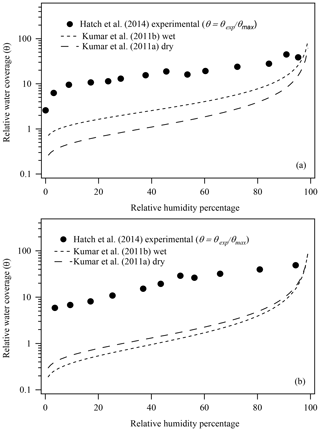Figure 1Comparison of previously reported experimental water adsorption isotherms ($\mathit{\theta }={\mathit{\theta }}_{\mathrm{exp}}/{\mathit{\theta }}_{\mathrm{max}}$) and FHH adsorption isotherms from FHH parameters determined from size-selected CCN measurements of (a) illite and (b) montmorillonite aerosol generated using wet or dry aerosol generation methods (Hatch et al., 2014; Kumar et al., 2011a, b).

3 FHH activation theory water adsorption analysis

The results discussed here are based on further assessment of experimental water adsorption measurements previously reported in the literature (Hatch et al., 2012, 2014). Hatch et al. (2012, 2014) reported water adsorption measurements on montmorillonite (SWy-2) and illite (IMt-1) clays obtained from the Clay Minerals Society's Source Clays Repository. Water adsorption was measured using a horizontal attenuated total reflectance Fourier transform infrared (HATR-FTIR) spectrometer with a humidified flow reactor. Details of experimental procedures and adsorbed water quantification can be found in the literature (Hatch et al., 2012, 2014). Water content as a function of RH was reported as a mass ratio of adsorbed water to dry mineral sample mass, ${m}_{{\mathrm{H}}_{\mathrm{2}}\mathrm{O}}/{m}_{\mathrm{sample}}$ (in ${g}_{{\mathrm{H}}_{\mathrm{2}}\mathrm{O}}/{g}_{\mathrm{sample}}\right)$, and was found to be in excellent agreement with previous gravimetric water content measurements on the same clays (Hatch et al., 2012; Schuttlefield et al., 2007b).

Traditionally, water content reported as ${m}_{{\mathrm{H}}_{\mathrm{2}}\mathrm{O}}/{m}_{\mathrm{sample}}$ is used to determine the relative surface coverage (θ) by first converting the mass ratio to an experimental coverage, θexp (molec. cm−2), using Eq. (4) (Tang et al., 2016).

$\begin{array}{}\text{(4)}& {\mathit{\theta }}_{\mathrm{exp}}=\frac{{m}_{{\mathrm{H}}_{\mathrm{2}}\mathrm{O}}}{{m}_{\mathrm{sample}}}\frac{{N}_{\mathrm{A}}}{{M}_{{\mathrm{H}}_{\mathrm{2}}\mathrm{O}}{A}_{\mathrm{BET}}},\end{array}$

where NA is Avogadro's number, ${M}_{{\mathrm{H}}_{\mathrm{2}}\mathrm{O}}$ is the molar mass of water, and ABET is the BET surface area using N2 as the adsorbate. The relative surface coverage is then determined by dividing θexp by a maximum coverage, θmax, or the maximum number of water molecules per centimeter squared that can be adsorbed to form a complete monolayer (ML) on the mineral surface. θmax is often approximated as 1×1015 molec. cm−2 or the inverse of the cross-sectional area of a water molecule, 1∕πr2, where r is the radius of a water molecule. This method for obtaining θ from the experimental mass ratio of adsorbed water has been used in previous studies (Hatch et al., 2014; Hudson et al., 2002; Schuttlefield et al., 2007a, b) and was recommended as the preferred technique in a recent review paper (Tang et al., 2016). However, obtaining θ in this way is based on assumptions that are not relevant to the systems studied and could introduce large uncertainties. For example, the water molecule is assumed to be spherical on a molecular scale. More significantly, θmax is calculated assuming a flat surface. That is, θmax represents the maximum number of spherical water molecules that can fit on a flat surface of 1 cm2. However, atmospheric mineral dust particles are widely known to exhibit significant surface microstructure and porosity, leading to a significantly larger surface area than that of a flat surface. Thus, the above method for obtaining θ from a mass ratio of adsorbed water can significantly overestimate θ, leading to erroneous FHH adsorption parameters upon fitting the FHH adsorption model to experimental water adsorption isotherms. The estimated θmax is expected to account, at least in part, for differences in FHH parameters and adsorption isotherms obtained from water adsorption and CCN activation measurements (Fig. 1).

More accurate θ values that account for the surface microstructure of the clay particles can be determined if the maximum ML water coverage is directly determined from experimental water adsorption data. The Brunauer–Emmett–Teller (BET) adsorption model is commonly applied to multilayer adsorption isotherms to determine a sample's specific surface area based on the amount (in volume) of adsorbate necessary to achieve ML coverage and the size of the adsorbate molecule. Equation (5) shows the linear form of the BET model (Brunauer et al., 1938).

$\begin{array}{}\text{(5)}& \frac{\frac{P}{{P}_{o}}}{\left(\mathrm{1}-\frac{P}{{P}_{o}}\right)V}=\frac{\mathrm{1}}{{V}_{{m}^{C}}}+\frac{\left(c-\mathrm{1}\right)}{{V}_{{m}^{C}}}\left(\frac{P}{{P}_{o}}\right)\end{array}$

In Eq. (5), $\frac{P}{{P}_{o}}$ represents RH, V is the measured volume (cm3) of surface adsorbed water, Vm is the volume (cm3) of water necessary to achieve ML coverage, and c is a constant that is related to the enthalpy of adsorption for any layer of adsorbed water. Vm and c can be determined by fitting experimental adsorption isotherms with Eq. (5) (Brunauer et al., 1938; Hatch et al., 2012). Since Vm is the volume equivalent of θmax, the relative surface coverage can be determined by $\mathit{\theta }=V/{V}_{m}$ as in Hung et al. (2015). BET analysis of water adsorption on illite and montmorillonite clays showed that ML water adsorption occurs at 0.065±0.032 and 0.063±0.036 g${}_{{\mathrm{H}}_{\mathrm{2}}\mathrm{O}}/{g}_{\mathrm{sample}}$, respectively (Hatch et al., 2012). The volume of adsorbed water necessary to achieve ML coverage can be calculated from these ML water content values expressed as mass ratios (Hatch et al., 2012) following Eq. (6).

$\begin{array}{}\text{(6)}& V=\frac{{m}_{{\mathrm{H}}_{\mathrm{2}}\mathrm{O}}}{{m}_{\mathrm{sample}}}\frac{{m}_{\mathrm{sample}}}{{D}_{{\mathrm{H}}_{\mathrm{2}}\mathrm{O}}}\end{array}$

In Eq. (6), msample is the mass (g) of sample, $\frac{{m}_{{\mathrm{H}}_{\mathrm{2}}\mathrm{O}}}{{m}_{\mathrm{sample}}}$ represents the experimental mass ratio of adsorbed water (${g}_{{\mathrm{H}}_{\mathrm{2}}\mathrm{O}}/{g}_{\mathrm{sample}}$), and ${D}_{{\mathrm{H}}_{\mathrm{2}}\mathrm{0}}$ is the density of water at room temperature (997.045 kg m−3) (Lide, 1995). Given illite and montmorillonite sample masses of 0.8 and 0.3 mg, Vm is calculated, to be $\mathrm{5.2}×{\mathrm{10}}^{-\mathrm{5}}$ and $\mathrm{1.9}×{\mathrm{10}}^{-\mathrm{5}}$ cm3, respectively, based on BET analysis of experimental water adsorption data (Hatch et al., 2012).

For comparison, Vm based on the estimated θmaxvalue of 1×1015 molec. cm−2 can be calculated using Eq. (7).

$\begin{array}{}\text{(7)}& \mathrm{Estimated}\phantom{\rule{0.25em}{0ex}}{V}_{m}=\frac{{\mathit{\theta }}_{\mathrm{max}}{M}_{{\mathrm{H}}_{\mathrm{2}}\mathrm{O}}{A}_{\mathrm{BET}}{m}_{\mathrm{sample}}}{{N}_{A}{D}_{{\mathrm{H}}_{\mathrm{2}}\mathrm{O}}}\end{array}$

In Eq. (7), ${M}_{{\mathrm{H}}_{\mathrm{2}}\mathrm{O}}$ is in kilograms per mole (kg mol−1), ${D}_{{\mathrm{H}}_{\mathrm{2}}\mathrm{0}}$ is in kilograms per cubic centimeter (kg cm−3), and ABET (using N2 as an adsorbate) is in centimeters squared per gram (cm2 g−1) (Hatch et al., 2012). A θmax of 1×1015 molec. cm−2 is equivalent to Vm values of $\mathrm{5.0}×{\mathrm{10}}^{-\mathrm{6}}$ and $\mathrm{2.3}×{\mathrm{10}}^{-\mathrm{6}}$ cm3 water for illite and montmorillonite clays, respectively. This is approximately an order of magnitude less adsorbed water at ML coverage than Vm values directly determined from experimental water adsorption data using BET analysis. Thus, previous studies that use θmax to calculate θ are overestimating the relative water coverage by up to an order of magnitude. This result is consistent with discrepancies in FHH curves determined based on previous water adsorption and CCN activation measurements illustrated in Fig. 1 and thus is likely to be a major source of the disagreement observed in the literature.

Using Eq. (6), V and θ, where $\mathit{\theta }=V/{V}_{m}$, were determined as a function of the relative humidity percentage based on previously reported water content mass ratios for illite and montmorillonite clays (Hatch et al., 2012). Figure 2 shows the calculated θ for (a) illite and (b) montmorillonite as a function of the relative humidity percentage based on obtaining Vm from BET analysis of experimental water adsorption data (Hatch et al., 2012). For comparison, adsorption curves calculated using Eq. (1) based on AFHH and BFHH parameters derived from CCN activation measurements of dry-generated illite and montmorillonite are also shown (Kumar et al., 2011a, b). In contrast to Fig. 1, which shows θ as calculated using the estimated θmax, Fig. 2 demonstrates that direct measurement of ML water content using BET analysis of the experimental data significantly enhances closure between adsorption isotherms derived from water adsorption and CCN activation measurements of microstructured clay minerals. As shown, the experimental adsorption curves of θ as a function of the relative humidity percentage are now in much better agreement with FHH adsorption curves based on FHH parameters from dry-generated illite and montmorillonite clay minerals, thus reducing the disagreement between these two methods (Hatch et al., 2014; Kumar et al., 2011a, b; Laaksonen et al., 2016; Tang et al., 2016).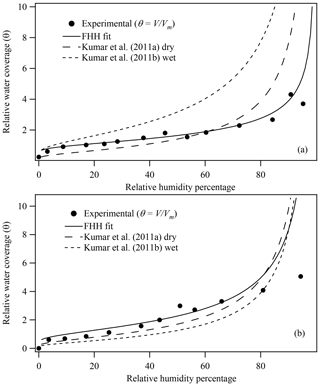Figure 2(a) Illite and (b) montmorillonite experimental water adsorption isotherms (Hatch et al., 2012) and associated FHH fit based on constrained FHH analysis, where the experimental θ was calculated as VVm and Vm was determined from BET analysis of adsorption isotherms. FHH adsorption isotherms from FHH parameters determined from size-selected CCN measurements of aerosol generated using wet or dry aerosol generation methods are also shown (Kumar et al., 2011a).

To obtain more accurate FHH adsorption parameters from the experimental water adsorption data for illite and montmorillonite clays shown in Fig. 2, Eq. (1) was rearranged to a linear relationship as shown in Eq. (8) (Tang et al., 2016).

$\begin{array}{}\text{(8)}& \mathrm{ln}\left[-\mathrm{ln}\left(s\right)\right]=\mathrm{ln}{A}_{\mathrm{FHH}}-{B}_{\mathrm{FHH}}\mathrm{ln}\mathit{\theta }\end{array}$

Linear regression analysis of ln[ln (s)] as a function of ln θ from 40 %–90 % RH allows for the determination of AFHH and BFHH (Hung et al., 2015; Tang et al., 2016). According to Hung et al. (2015), constraining the FHH adsorption model fit to a limited range of high RH values avoids uncertainties due to assumptions inherent in the FHH adsorption theory, as the fit should be limited to the multilayer water adsorption regime. Importantly, the FHH adsorption model assumes that particles are spherical, of a single universal diameter, and have a smooth surface and that water is uniformly distributed (Hill, 1952). These assumptions are problematic when applied to adsorption measurements on bulk, polydisperse mineral dust particles which are known to be irregularly shaped and porous. Constraining the fit to higher RH values helps avoid surface porosity effects on the resulting FHH adsorption parameters. However, swelling clay minerals, such as montmorillonite, are problematic as the multilayer adsorption regime begins at higher RH values. For example, previous studies have shown that the multilayer adsorption regime begins at ∼70 % RH for montmorillonite clay (Cases et al., 1992; Mooney et al., 1952), and thus the FHH fit should be constrained from 70 % to 90 % RH. Unfortunately, the limited number of data at high RH values precludes the feasibility of fitting the FHH model over this smaller range of RH values. Thus, the FHH fit parameters reported here for montmorillonite suffer from uncertainty due to the swelling action of smectite clays.

Figure 3 shows the FHH adsorption theory analysis of experimental water adsorption on illite and montmorillonite clays based on a constrained FHH fit as described above and θ calculated as VVm, where Vm was directly measured from the experimental water adsorption data using BET analysis. The closed circles represent the data fit to the FHH equation (Eq. 8). Resulting AFHH and BFHH values are reported in Table 1. For comparison, FHH parameters previously reported in the literature that were determined using other methods are also reported. FHH parameters from analysis of previously reported water adsorption data (Hatch et al., 2014) assuming $\mathit{\theta }={\mathit{\theta }}_{\mathrm{exp}}/{\mathit{\theta }}_{\mathrm{max}}$, and water adsorption (montmorillonite only) analyzed based on $\mathit{\theta }=V/{V}_{m}$ are reported. Table 1 also shows the constrained FHH parameters using a surface area and porosity measurement system (Hung et al., 2015) as well as experimental CCN activation measurements of wet- and dry-generated clay minerals (Kumar et al., 2011a, b). In general, the FHH parameters from water adsorption measurements using the method reported here agree more closely with CCN activation-derived FHH parameters compared to those reported previously for the same sample (Hatch et al., 2014). Using Eq. (3), illite and montmorillonite water adsorption isotherms (Fig. 2, solid lines) were calculated based on AFHH and BFHH values determined here (Table 1, this study). As shown in Fig. 2, the FHH curves based on analysis of experimental water adsorption appear to fit the experimental data very well and the adsorption isotherms show significantly improved agreement with FHH isotherms calculated from CCN activation measurements of the same clays. Although significant advances toward closure between FHH parameters from water adsorption and CCN activation measurements are demonstrated here, differences remain between FHH parameters determined using different methods. Thus, continued efforts to identify improved agreement between FHH parameters from water adsorption and CCN activation measurements are warranted.

Table 1AFHH and BFHH determined from water adsorption and CCN activation measurements of dry- and wet-generated illite and montmorillonite clays.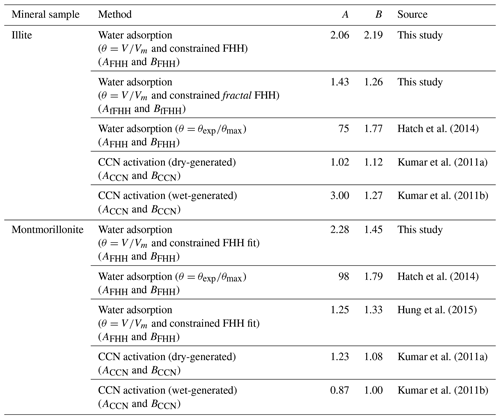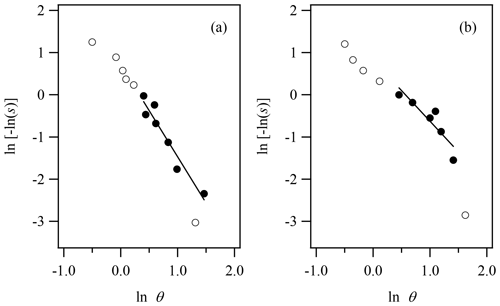Figure 3FHH analysis of experimental (a) illite and (b) montmorillonite water adsorption data in which θ was calculated as VVm and Vm was determined from BET analysis. All data (open circles) are shown and the region of the constrained FHH fit (line) is represented by closed circles from 40 % to 90 % RH.

Despite the improved agreement observed upon direct measurement of ML water content and constraining the FHH theory fits to the multilayer adsorption regime, small discrepancies in isotherm structure remain, as shown in Fig. 2. Structural isotherm discrepancies between the two methods are potentially a result of surface heterogeneity and microstructural differences in how space-filling by water adsorption occurs between bulk and aerosol measurements. A recent paper (Laaksonen et al., 2016) suggests that the surface fractal dimension influences water adsorption on insoluble surfaces and thus could help achieve closure between water adsorption and CCN activation measurements. In an effort to account for the observed differences in isotherm structure, the fractal FHH adsorption theory was used to demonstrate the effects of correcting the adsorption isotherm using the surface fractal dimension, D, as discussed in Laaksonen et al. (2016). The fractal FHH adsorption theory is expressed as

$\begin{array}{}\text{(9)}& \mathrm{ln}S=-{A}_{\mathrm{fFHH}}{k}^{-{B}_{\mathrm{fFHH}}}{\left(\frac{V}{{V}_{m}}\right)}^{\frac{-{B}_{\mathrm{fFHH}}}{\mathrm{3}\text{-D}}},\end{array}$

according to Laaksonen et al. (2016), where k is a proportionality constant that is equal to unity in the case of a smooth surface and AfFHH and BfFHH are the fractal FHH adsorption parameters. D can vary from a value of 2 to 3, where a fractal dimension of 2 represents a completely smooth surface and a value of three represents a surface that fills its space. Here, fractal FHH analysis is restricted to illite clay adsorption data due to the limited data available for montmorillonite in the higher RH range of the multilayer adsorption regime for swelling clays.

The inset of Fig. 4 shows the fractal FHH adsorption theory fit to the experimental water adsorption results for illite clay, again constrained from 40 % to 90 % RH within the multilayer adsorption regime for illite. The resulting fractal FHH adsorption parameters are reported in Table 1 and the resulting k and D parameters are found to be 0.97 and 2.35, respectively. For comparison, Laaksonen et al. (2016) obtained surface fractal dimensions for illite clay ranging from 2.39 to 2.69. Notably, the surface fractal dimension results reported by Laaksonen et al. (2016) are based on clays obtained from different sources and heat treated prior to measurements and thus cannot be directly compared to results reported here. In fractal FHH theory, AFHH and BFHH are represented by ${A}_{\mathrm{fFHH}}{k}^{-{B}_{\mathrm{fFHH}}}$ and $\frac{-{B}_{\mathrm{fFHH}}}{\text{3-D}}$, respectively. Applying the resulting k and D values, fractal FHH adsorption analysis gives AFHH and BFHH adsorption parameters of 1.48 and 1.94 for illite clay.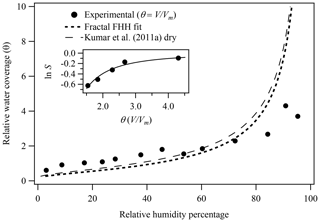Figure 4Illite experimental water adsorption isotherm (Hatch et al., 2012) and associated fractal FHH isotherm, where the experimental θ was calculated as VVm and Vm was determined from BET analysis of adsorption isotherm. FHH adsorption isotherms from FHH parameters determined from size-selected CCN measurements of dry-generated illite clay are also shown (Kumar et al., 2011a). The inset shows the constrained fractal FHH isotherm fit to the experimental water adsorption measurements.

Figure 4 also shows the adsorption isotherm generated based on the fractal FHH adsorption parameters obtained compared to the FHH adsorption isotherm from FHH parameters determined from CCN measurements of dry-generated illite clay reported by Kumar et al. (2011a). As shown in Table 1 and Fig. 4, the fractal FHH adsorption analysis results in a modeled isotherm based on experimental water adsorption measurements that exhibit significantly improved agreement with the isotherm obtained from CCN activation measurements of dry-generated illite. This demonstrates that correcting for the surface fractal dimension in experimental water adsorption measurements helps bring closure between aerosol and bulk methods for measuring the uptake of water on insoluble surfaces.

4 Concluding remarks

Experimentally determined FHH water adsorption parameters remain the largest source of uncertainty in assessing the role of insoluble aerosol on liquid cloud formation and the indirect climate effect (Karydis et al., 2012). Thus, accurate measurements of FHH adsorption parameters are necessary to reduce this uncertainty. However, Hatch et al. (2014) have shown that FHH parameters derived from water adsorption measurements (Eq. 1) can differ significantly from values based on FHH-AT analysis of experimental CCN activation measurements (Eq. 2) of the same mineral components (Kumar et al., 2011a, b). The work reported here shows that (1) improved fitting procedures, (2) direct measurement of ML water content, and (3) applying the fractal FHH adsorption model to the experimental water adsorption measurements account for surface microstructure effects and are necessary to obtain closure between experimental water adsorption and CCN-derived FHH parameters.

To assess the improved agreement between FHH adsorption parameters (Table 1) from water adsorption measurements and FHH-AT analysis of dry-generated mineral aerosol CCN activation measurements, a percent difference can be calculated. Results for illite clay indicate that the improved FHH analysis methods described here, including application of the fractal FHH adsorption isotherm, reduce the percentage difference in AFHH from the value determined based on an FHH-AT assessment of dry-generated aerosol CCN activation from 195 % to ∼33 % difference (Hatch et al., 2014; Kumar et al., 2011a). Thus, as the AFHH value governs the overall extent of water coverage, the significant reduction in experimental θ based on direct measurements of ML water content from BET analysis of water adsorption is driving improved accuracy of AFHH values. Results also demonstrate that the agreement between BFHH values from water adsorption and CCN activation measurements of illite clay also improved (from 45 % to 12 % difference from dry-generated aerosol CCN measurements), particularly upon application of the fractal FHH adsorption model, thereby accounting for space-filling effects of the insoluble surface. Thus, improved agreement between bulk water adsorption and aerosol CCN activation, along with a refined focus on the multilayer adsorption regime and accounting for surface microstructure through direct measurement of the ML water content and application of the fractal FHH adsorption isotherm, demonstrates that closure can be achieved.

Data availability

The data used in this publication are available to the community and can be accessed by request to the corresponding author.

Author contributions

MAC, RM, and MJC contributed to data collection and RT, AG, and CH performed all associated data analysis. The paper was written through contributions from all authors. All authors have given approval to the final version of the paper.

Competing interests

The authors declare that they have no conflict of interest.

Acknowledgements

In addition to external funding, this project was supported by the Hendrix College Odyssey Program and the Hendrix College Morris and Ann Henry Odyssey Professorship.

Financial support

This research has been supported by the National Science Foundation, Division of Atmospheric and Geospace Sciences (grant no. 1755606) and the Arkansas Space Grant Consortium (grant no. NNX10AL28H).

Review statement

This paper was edited by Hang Su and reviewed by Ari Laaksonen and one anonymous referee.

References

Bangert, M., Nenes, A., Vogel, B., Vogel, H., Barahona, D., Karydis, V. A., Kumar, P., Kottmeier, C., and Blahak, U.: Saharan dust event impacts on cloud formation and radiation over Western Europe, Atmos. Chem. Phys., 12, 4045–4063, https://doi.org/10.5194/acp-12-4045-2012, 2012.

Brunauer, S., Emmett, P. H., and Teller, E.: Adsorption of gases in multimolecular layers, J. Am. Chem. Soc., 60, 309–319, 1938.

Cases, J. M., Berend, I., Besson, G., Francois, M., Uriot, J. P., Thomas, F., and Poirier, J. E.: Mechanism of adsorption and desorption of water vapor by homoionic montmorillonite. 1. The sodium-exchanged form, Langmuir, 8, 2730–2739, 1992.

Creamean, J. M., Suski, K. J., Rosenfeld, D., Cazorla, A., DeMott, P. J., Sullivan, R. C., White, A. B., Ralph, F. M., Minnis, P., Comstock, J. M., Tomlinson, J. M., and Prather, K. A.: Dust and biological aerosols from the Sahara and Asia influence precipitation in the western U.S, Science, 339, 1572–1578, https://doi.org/10.1126/science.1227279, 2013.

Cwiertny, D. M., Young, M. A., and Grassian, V. H.: Chemistry and photochemistry of mineral dust aerosol, Annu. Rev. Phys. Chem., 59, 27–51, https://doi.org/10.1146/annurev.physchem.59.032607.093630, 2008.

Forster, P., Ramaswamy, V., Artaxo, P., Berntsen, T., Betts, R., Fahey, D. W., Haywood, J., Lean, J., Lowe, D. C., Myhre, G. Nganga, J., Prinn, R., Raga, G., Schulz, M., and Van Dorland, R.: Changes in atmospheric constituents and in radiative forcing, Climate Change 2007: The Physical Science Basis. Contribution of Working Group I to the Fourth Assessment Report of the Intergovernmental Panel on Climate Change, Cambridge University Press, 210–215, 2007.

Ginoux, P., Prospero, J. M., Gill, T. E., Hsu, N. C., and Zhao, M.: Global-scale attribution of anthropogenic and natural dust sources and their emission rates based on MODIS Deep Blue aerosol products, Rev. Geophys., 50, RG3005, https://doi.org/10.1029/2012rg000388, 2012.

Hatch, C. D., Wiese, J. S., Crane, C. C., Harris, K. J., Kloss, H. G., and Baltrusaitis, J.: Water adsorption on clay minerals as a function of relative humidity: Application of BET and Freundlich adsorption models, Langmuir, 28, 1790–1803, 2012.

Hatch, C. D., Greenaway, A. L., Christie, M. J., and Baltrusaitis, J.: Water adsorption constrained Frenkel–Halsey–Hill adsorption activation theory: Montmorillonite and illite, Atmos. Environ., 87, 26–33, https://doi.org/10.1016/j.atmosenv.2013.12.040, 2014.

Herich, H., Tritscher, T., Wiacek, A., Gysel, M., Weingartner, E., Lohmann, U., Baltensperger, U., and Cziczo, D. J.: Water uptake of clay and desert dust aerosol particles at sub- and super-saturated water vapor conditions, Phys. Chem. Chem. Phys., 11, 7804–7809, 2009.

Hill, T. L.: Theory of Physical Adsorption, in: Advances in Catalysis, edited by: Frankenburg, W. G., Komarewsky, V. I., and Rideal, E. K., Academic Press, 211–258, 1952.

Hudson, P. K., Zondlo, M. A., and Tolbert, M. A.: The interaction of methanol, acetone, and acetaldehyde with ice and nitric acid-doped ice: Implications for cirrus clouds, J. Phys. Chem. A, 106, 2882–2888, 2002.

Hung, H.-M., Wang, K.-C., and Chen, J.-P.: Adsorption of nitrogen and water vapor by insoluble particles and the implication on cloud condensation nuclei activity, J. Aerosol Sci., 86, 24–31, https://doi.org/10.1016/j.jaerosci.2015.04.002, 2015.

IPCC: Climate Change 2013: The Physical Science Basis, Cambridge University Press, 2013.

Karanasiou, A., Moreno, N., Moreno, T., Viana, M., de Leeuw, F., and Querol, X.: Health effects from Sahara dust episodes in Europe: literature review and research gaps, Environ. Int., 47, 107–114, https://doi.org/10.1016/j.envint.2012.06.012, 2012.

Karydis, V. A., Kumar, P., Barahona, D., Sokolik, I. N., and Nenes, A.: On the effect of dust particles on global cloud condensation nuclei and cloud droplet number, J. Geophys. Res., 116, D23204, https://doi.org/10.1029/2011JD016283, 2011.

Karydis, V. A., Capps, S. L., Russell, A. G., and Nenes, A.: Adjoint sensitivity of global cloud droplet number to aerosol and dynamical parameters, Atmos. Chem. Phys., 12, 9041–9055, https://doi.org/10.5194/acp-12-9041-2012, 2012.

Koehler, K. A., Kreidenweis, S. M., DeMott, P. J., Petters, M. D., Prenni, A. J., and Carrico, C. M.: Hygroscopicity and cloud droplet activiation of mineral dust aerosol, Geophys. Res. Lett., 36, 1–5, 2009.

Kumar, P., Nenes, A., and Sokolik, I. N.: Importance of adsorption for CCN activity and hygroscopic properties of mineral dust aerosol, Geophys. Res. Lett., 36, L24804, https://doi.org/10.1029/2009GL040827, 2009a.

Kumar, P., Sokolik, I. N., and Nenes, A.: Parameterization of cloud droplet formation for global and regional models: including adsorption activation from insoluble CCN, Atmos. Chem. Phys., 9, 2517–2532, https://doi.org/10.5194/acp-9-2517-2009, 2009b.

Kumar, P., Sokolik, I. N., and Nenes, A.: Measurements of cloud condensation nuclei activity and droplet activation kinetics of fresh unprocessed regional dust samples and minerals, Atmos. Chem. Phys., 11, 3527–3541, https://doi.org/10.5194/acp-11-3527-2011, 2011a.

Kumar, P., Sokolik, I. N., and Nenes, A.: Cloud condensation nuclei activity and droplet activation kinetics of wet processed regional dust samples and minerals, Atmos. Chem. Phys., 11, 8661–8676, https://doi.org/10.5194/acp-11-8661-2011, 2011b.

Laaksonen, A.: A unifying model for adsorption and nucleation of vapors on solid surfaces, J. Phys. Chem. A, 119, 3736–3745, https://doi.org/10.1021/acs.jpca.5b00325, 2015.

Laaksonen, A., Malila, J., Nenes, A., Hung, H. M., and Chen, J. P.: Surface fractal dimension, water adsorption efficiency, and cloud nucleation activity of insoluble aerosol, Sci. Rep.-UK, 6, 25504, https://doi.org/10.1038/srep25504, 2016.

Lide, D. R. (Ed.): CRC Handbook of Chemistry and Physics: A ready-reference book of chemical and physical data, CRC Press, Boca Raton, FL, 1995.

Mahowald, N. M., Ballantine, J. A., Feddema, J., and Ramankutty, N.: Global trends in visibility: implications for dust sources, Atmos. Chem. Phys., 7, 3309–3339, https://doi.org/10.5194/acp-7-3309-2007, 2007.

Manktelow, P. T., Carslaw, K. S., Mann, G. W., and Spracklen, D. V.: The impact of dust on sulfate aerosol, CN and CCN during an East Asian dust storm, Atmos. Chem. Phys., 10, 365–382, https://doi.org/10.5194/acp-10-365-2010, 2010.

Mooney, R. W., Keenan, A. G., and Wood, L. A.: Adsorption of water vapor by Montmorillonite. II. Effect of exchangable ions and lattice swelling as measured by X-Ray diffraction, J. Am. Chem. Soc., 74, 1371–1374, 1952.

Pringle, K. J., Tost, H., Pozzer, A., Pöschl, U., and Lelieveld, J.: Global distribution of the effective aerosol hygroscopicity parameter for CCN activation, Atmos. Chem. Phys., 10, 5241–5255, https://doi.org/10.5194/acp-10-5241-2010, 2010.

Prospero, J. M. and Lamb, P. J.: African droughts and dust transport to the Caribbean: climate change implications, Science, 302, 1024–1027, https://doi.org/10.1126/science.1089915, 2003.

Pruppacher, H. R. and Klett, J. D.: Microphysics of clouds and precipitation, Reidel Publishing Company, Dordrecht, 1980.

Schuttlefield, J., Al-Hosney, H., Zachariah, A., and Grassian, V. H.: Attenuated total reflection fourier transform infrared spectroscopy to investigate water uptake and phase transitions in atmospherically relevant particles, Appl. Spectrosc., 61, 283–292, https://doi.org/10.1366/000370207780220868, 2007a.

Schuttlefield, J. D., Cox, D., and Grassian, V. H.: An investigation of water uptake on clays minerals using ATR-FTIR spectroscopy coupled with quartz crystal microbalance measurements, J. Geophys. Res.-Atmos., 112, D21303, https://doi.org/10.1029/2007JD008973, 2007b.

Smoydzin, L., Teller, A., Tost, H., Fnais, M., and Lelieveld, J.: Impact of mineral dust on cloud formation in a Saharan outflow region, Atmos. Chem. Phys., 12, 11383–11393, https://doi.org/10.5194/acp-12-11383-2012, 2012.

Sorjamaa, R. and Laaksonen, A.: The effect of H2O adsorption on cloud drop activation of insoluble particles: a theoretical framework, Atmos. Chem. Phys., 7, 6175–6180, https://doi.org/10.5194/acp-7-6175-2007, 2007.

Stevens, B. and Feingold, G.: Untangling aerosol effects on clouds and precipitation in a buffered system, Nature, 461, 607–613, https://doi.org/10.1038/nature08281, 2009.

Tang, M., Cziczo, D. J., and Grassian, V. H.: Interactions of Water with Mineral Dust Aerosol: Water Adsorption, Hygroscopicity, Cloud Condensation, and Ice Nucleation, Chem. Rev., 116, 4205–4259, https://doi.org/10.1021/acs.chemrev.5b00529, 2016.

Textor, C., Schulz, M., Guibert, S., Kinne, S., Balkanski, Y., Bauer, S., Berntsen, T., Berglen, T., Boucher, O., Chin, M., Dentener, F., Diehl, T., Easter, R., Feichter, H., Fillmore, D., Ghan, S., Ginoux, P., Gong, S., Grini, A., Hendricks, J., Horowitz, L., Huang, P., Isaksen, I., Iversen, I., Kloster, S., Koch, D., Kirkevåg, A., Kristjansson, J. E., Krol, M., Lauer, A., Lamarque, J. F., Liu, X., Montanaro, V., Myhre, G., Penner, J., Pitari, G., Reddy, S., Seland, Ø., Stier, P., Takemura, T., and Tie, X.: Analysis and quantification of the diversities of aerosol life cycles within AeroCom, Atmos. Chem. Phys., 6, 1777–1813, https://doi.org/10.5194/acp-6-1777-2006, 2006.

Zhu, T., Shang, J., and Zhao, D.: The roles of heterogeneous chemical processes in the formation of an air pollution complex and gray haze, Sci. China Chem., 54, 145–153, https://doi.org/10.1007/s11426-010-4181-y, 2011.